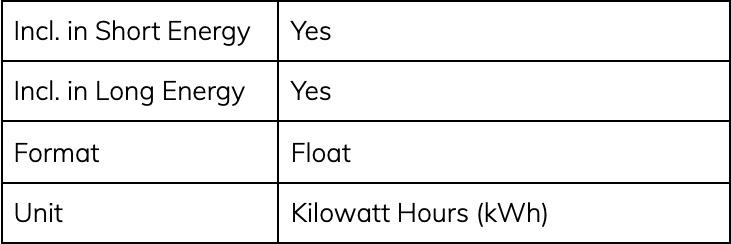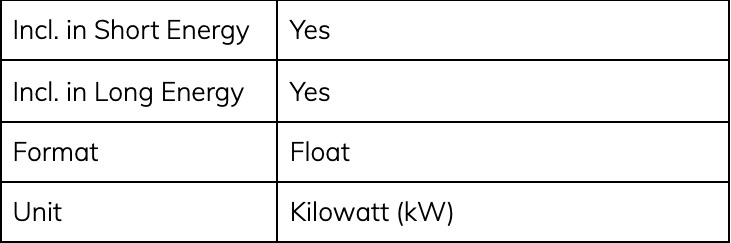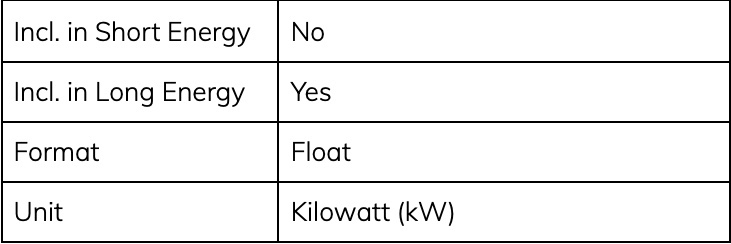# What data does the device capture?

## For the public to understand what data that the Auditor is capturing

#### Short Energy

Short Energy is data captured every 5 seconds and sent every 30 seconds on a “best efforts” basis. This is useful for real-time data visualisation or analysis. This could be considered akin to the MQTT Quality of Service (QoS) level 0—at most once.

#### Long Energy

Long Energy is a 5-minute aggregation of the 5-second data that is sent on the 5-minute boundary. If the device is offline, this data is logged locally for a period, and when the device comes back online we send the data on a FIFO basis to our servers. This provides a stronger QoS level 1—at least once.

The Auditor is an energy monitoring device that captures a wide variety of data from a site and the circuits that it is monitoring including:

• Active/Real Energy
• Reactive Energy
• Voltage
• Current
• Frequency

These foundations that are captured and reported by the device can be used to calculate a wider range of data points (such as reactive power, power factor) and be converted into different units (e.g. Joules to kWh).

### Data available via the API

Our REST API provides options to get the raw data from our devices, as well as providing options to calculate common conversions for key fields.

#### eReal

Active/real energy.#### eRealPositive

Positive active/real energy. Over the Long Energy period there may be points of negative energy (e.g. drawing energy from the grid) that are masked in the eReal value for the same period. For example, if the overall total is negative (for example if solar generation results in an overall export for the period). eRealPositive enables you to determine the total positive flows during the period.#### eRealNegative

Negative active/real energy. Over the Long Energy period there may be points of negative energy (e.g. exports to the grid) that are "hidden" in the eReal value for the period if the overall total is positive. eRealNegative enables you to determine the total negative flows during the period.#### eReactive

Reactive energy.#### eReactivePositive

Positive reactive energy.#### eReactiveNegative

Negative reactive energy.#### vRMS

Volts Root Mean Square (RMS).#### vRMSMin

Minimum Volts RMS. As voltage is an instantaneous value, only the minimum and maximum that occurred within the period are reported for Long Energy.#### vRMSMax

Maximum Volts RMS. As voltage is an instantaneous value, only the minimum and maximum that occurred within the period are reported for Long Energy.#### iRMS

Current Root Mean Square (RMS).#### iRMSMin

Minimum Current RMS. As current is an instantaneous value, only the minimum and maximum that occurred within the period are reported for Long Energy.#### iRMSMax

Maximum Current RMS. As current is an instantaneous value, only the minimum and maximum that occurred within the period are reported for Long Energy.#### frequency

Voltage Frequency. Measured off P1.#### eRealKwh

Real Energy converted from Joules to kilowatt-hours.#### eRealPositiveKwh

Positive Real Energy converted from Joules to kilowatt-hours.#### eRealNegativeKwh

Negative Real Energy converted from Joules to kilowatt-hours.#### eReactiveKwh

Reactive Energy converted from Joules to kilowatt-hours.#### eReactivePositiveKwh

Positive Reactive Energy converted from Joules to kilowatt-hours.#### eReactiveNegativeKwh

Negative Reactive Energy converted from Joules to kilowatt-hours.#### pRealKw

Real Energy converted from Joules to kilowatts. Note this is a conversion of energy to power, thus the prefix changes to p.#### pRealPositiveKw

Positive Real Energy converted from Joules to kilowatts. Note this is a conversion of energy to power, thus the prefix changes to p.#### pRealNegativeKw

Negative Real Energy converted from Joules to kilowatts. Note this is a conversion of energy to power, thus the prefix changes to p.#### pReactiveKw

Reactive Energy converted from Joules to kilowatts. Note this is a conversion of energy to power, thus the prefix changes to p.#### pReactivePositiveKw

Positive Reactive Energy converted from Joules to kilowatts. Note this is a conversion of energy to power, thus the prefix changes to p.#### pReactiveNegativeKw

Negative Reactive Energy converted from Joules to kilowatts. Note this is a conversion of energy to power, thus the prefix changes to p.#### powerFactor

The calculated power factor for the channel.### Got questions?

Let us know by filling this form.